## Coin Tossing

An idealized coin consists of a circular disk of zero thickness which, when thrown in the air and allowed to fall, will rest with either side face up (heads'' H or tails'' T) with equal probability. A coin is therefore a two-sided Die. A coin toss corresponds to a Bernoulli Distribution with. Despite slight differences between the sides and Nonzero thickness of actual coins, the distribution of their tosses makes a good approximation to aBernoulli Distribution.

There are, however, some rather counterintuitive properties of coin tossing. For example, it is twice as likely that the triple TTH will be encountered before THT than after it, and three times as likely that THH will precede HTT. Furthermore, it is six times as likely that HTT will be the first of HTT, TTH, and TTT to occur (Honsberger 1979). More amazingly still, spinning a penny instead of tossing it results in heads only about 30% of the time (Paulos 1995).

Letbe the probability that no Run of three consecutive heads appears inindependent tosses of a Coin. The following table gives the first few values of.0 1 1 1 2 1 3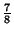45Feller (1968, pp. 278-279) proved that(1)

where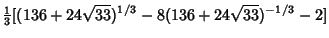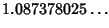(2)

and(3)

The corresponding constants for a Run ofheads are, the smallest Positive Root of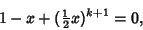(4)

and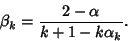(5)

These are modified for unfair coins withandto, the smallest Positive Root of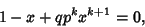(6)

and(7)

(Feller 1968, pp. 322-325).

References

Feller, W. An Introduction to Probability Theory and Its Application, Vol. 1, 3rd ed. New York: Wiley, 1968.

Finch, S. Favorite Mathematical Constants.'' http://www.mathsoft.com/asolve/constant/feller/feller.html

Ford, J. How Random is a Coin Toss?'' Physics Today 36, 40-47, 1983.

Honsberger, R. Some Surprises in Probability.'' Ch. 5 in Mathematical Plums (Ed. R. Honsberger). Washington, DC: Math. Assoc. Amer., pp. 100-103, 1979.

Keller, J. B. The Probability of Heads.'' Amer. Math. Monthly 93, 191-197, 1986.

Paulos, J. A. A Mathematician Reads the Newspaper. New York: BasicBooks, p. 75, 1995.

Peterson, I. Islands of Truth: A Mathematical Mystery Cruise. New York: W. H. Freeman, pp. 238-239, 1990.

Spencer, J. Combinatorics by Coin Flipping.'' Coll. Math. J., 17, 407-412, 1986.